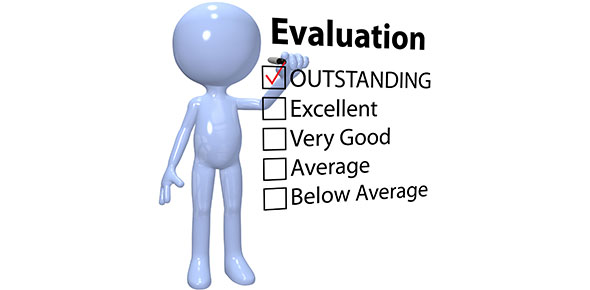# Are You Smarter Then A Fourth Grader?

Approved & Edited by ProProfs Editorial Team
The editorial team at ProProfs Quizzes consists of a select group of subject experts, trivia writers, and quiz masters who have authored over 10,000 quizzes taken by more than 100 million users. This team includes our in-house seasoned quiz moderators and subject matter experts. Our editorial experts, spread across the world, are rigorously trained using our comprehensive guidelines to ensure that you receive the highest quality quizzes.
| By Mickey111
M
Mickey111
Community Contributor
Quizzes Created: 1 | Total Attempts: 94
Questions: 9 | Attempts: 94SettingsYou will be asked questions, the subjects will be math and spelling. . . GOOD LUCK

• 1.

### WHAT IS 59 DIVIDED BY 7?

• A.

8

• B.

7 REMAINDER 1

• C.

8 REMAINDER 3

• D.

7

• E.

8 REMAINDER 2

C. 8 REMAINDER 3
Explanation
When 59 is divided by 7, the quotient is 8 and the remainder is 3. This means that 59 can be divided evenly by 7, resulting in 8 whole parts, with 3 remaining.

Rate this question:

• 2.

### HOW DO YOU SPELL THE BACKWARDS WORD OF RACECAR?

• A.

RCAECRA

• B.

RACECAR

• C.

CARRACE

• D.

RACECAC

• E.

CACRACE

B. RACECAR
Explanation
The given question asks for the spelling of the word "RACECAR" spelled backwards. The correct answer is "RACECAR" itself, as it remains the same when spelled backwards.

Rate this question:

• 3.

### WHAT IS 12X12?

• A.

124

• B.

145

• C.

125

• D.

112

• E.

144

E. 144
Explanation
The correct answer is 144 because 12 multiplied by 12 equals 144.

Rate this question:

• 4.

### WHAT IS 7X9+8+5?

• A.

98

• B.

76

• C.

55

• D.

72

• E.

88

B. 76
Explanation
To find the value of 7x9+8+5, we first perform the multiplication operation, which gives us 63. Then, we add 8 and 5 to get 16. Finally, we add 63 and 16, resulting in 79. Therefore, the correct answer is 76.

Rate this question:

• 5.

### HOW DO YOU SPELL PINEAPPLE WITHOUT THE APPLE PART?

• A.

PINE

• B.

APLE

• C.

APPLE

• D.

YOU CAN'T!

• E.

PIN

A. PINE
Explanation
The question asks for the correct spelling of "pineapple" without the "apple" part. The correct answer is "pine" because it is the remaining part of the word after removing "apple."

Rate this question:

• 6.

### IS 1,000000 A EVEN NUMBER?

• A.

NO

• B.

KINDA

• C.

YES

• D.

I DON'T KNOW

• E.

MAYBE

C. YES
Explanation
The given correct answer is "YES" because an even number is divisible by 2 without leaving a remainder. In this case, the number 1,000000 can be divided by 2, resulting in 500,000. Therefore, it is an even number.

Rate this question:

• 7.

### WHICH ONE IS A COMPLETE SENTENCE?

• A.

It is almost my birthday

• B.

HI

• C.

What is that?

• D.

GOOD NIGHT MOM I MISS YOU!

• E.

COOL

D. GOOD NIGHT MOM I MISS YOU!
Explanation
The correct answer is "GOOD NIGHT MOM I MISS YOU!" because it is the only option that forms a complete sentence. It includes a subject ("I"), a verb ("miss"), and expresses a complete thought. The other options are either incomplete phrases or do not form a grammatically correct sentence.

Rate this question:

• 8.

### THERE WAS 76 COOKIES 2 PEOLE ATE 7 AND THEN BOUGHT TWO MORE HOW MANY COOKIES WERE THERE IN ALL?

• A.

71

• B.

62

• C.

0

• D.

67

• E.

77

A. 71
Explanation
The question states that there were initially 76 cookies. Two people ate 7 cookies, which leaves 69 cookies. Then, they bought two more cookies, resulting in a total of 71 cookies.

Rate this question:

• 9.

### HOW MANY SYLLABLES ARE THERE FOR THANKSGIVING?

• A.

2

• B.

4

• C.

1

• D.

12

• E.

3

E. 3
Explanation
The word "Thanksgiving" has three syllables. The syllables are "Thanks-giv-ing".

Rate this question:

Related TopicsBack to top